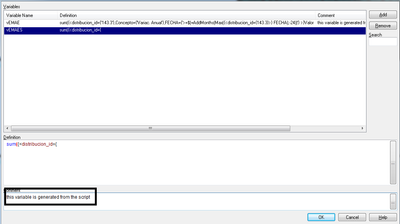# QlikView App Dev

Discussion Board for collaboration related to QlikView App Development.

Announcements
Support Case Portal has moved to Qlik Community! Read the FAQs to start exploring Support resources.
cancel
Showing results for
Did you mean:Creator III

## Expressions as variables. Error

Hello dear, I leave the following query

Why when I add an expression as a variable in the script, does it give me an error and if I do it from the variable panel is it ok?
The inconvenience I have with the expressions that contain set analysis, is it possible to do it?

Issue:Any suggestion?

Thanks!

1 Solution

Accepted SolutionsCreator III
Author

I found an interesting solution:
with this script you can load expressions from excel, since there are no problems with single quotes, it is also much easier to administer

Variable Table:
Expression
DESDE
[C: \ Nube \ Wicham \ EQonomia \ Variables.xlsx]
(ooxml, embedded labels, table is Sheet1);

let CantVariables = NoOfRows ('Variable Table');
for i = 0 to (CantVariables-1)
let vVariable = Peek ('Variable', i, 'TableVariables');
let \$ (vVariable) = Peek ('Expression', i, 'TableVariables');
NEXT

Thanks both!

4 RepliesMVP

It would help if you posted the part of the script that generated that. Most of use can't mind-read qlikview documents on other peoples computers.
What is going wrong are the single quotes. You need to either escape using double single quotes or chr(39) or another placeholder character that you later replace with the single quote

SET vMyVar = sum({<B={ @C@ }>} A);
LET vMyVar = Replace(\$(vMyVar), '@', chr(39));

But you're not done yet because \$(...something...) will be evaluated in the script too and you don't want that. So you can use the replace trick again

LET vMyVar = 'sum({<B={ @C@ }, D={"<=%(=max(E))"}>} A)';
LET vMyVar = Replace(Replace(\$(vMyVar), '@', chr(39)),'%','\$');

talk is cheap, supply exceeds demandCreator

the problem is the single quote which tells the script that it is at the start or the end of a piece of text, so you need to swap that for a chr() reference such as:

VariableName = 'Sum({\$<DistributionID={' & chr(39) & '143.3 etc etc etc

It can become quite long winded doing this in the script, but its the only way I've found to make it work.Creator III
Author
here it's how i load the variable in the script:

LET vEMAES ='sum({<distribucion_id={'143.3'},Concepto={'Variac. Anual'},FECHA={'>=\$(=AddMonths(Max({<distribucion_id={143.3}>} FECHA),-24))'} >}Valores)';Creator III
Author

I found an interesting solution:
with this script you can load expressions from excel, since there are no problems with single quotes, it is also much easier to administer

Variable Table:
Expression
DESDE
[C: \ Nube \ Wicham \ EQonomia \ Variables.xlsx]
(ooxml, embedded labels, table is Sheet1);

let CantVariables = NoOfRows ('Variable Table');
for i = 0 to (CantVariables-1)
let vVariable = Peek ('Variable', i, 'TableVariables');
let \$ (vVariable) = Peek ('Expression', i, 'TableVariables');
NEXT

Thanks both!Community Browser